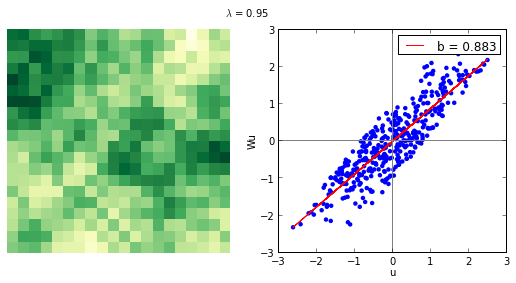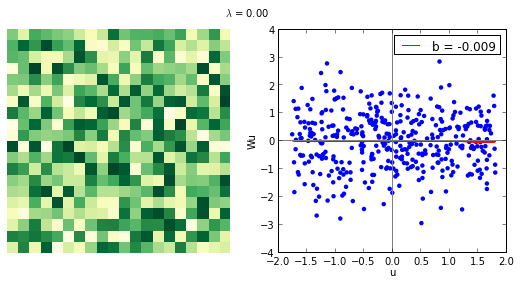In :
import pysal as ps
import numpy as np
import matplotlib.pyplot as plt
from scipy.linalg import inv

In :
%matplotlib inline


# Interactive spatial autocorrelation¶

This notebook illustrates the concept of spatial autocorrelation using the new interactivity in IPython. The data generating process (DGP) considered here is the following:

1. $u = \lambda Wu + \epsilon$

2. $u - \lambda Wu = \epsilon$

3. $u (I - \lambda W) = \epsilon$

4. $u = (I - \lambda W)^{-1} \epsilon$

Where u is a vector spatially autocorrelated, W is a spatial weights matrix as you could created with PySAL, and $\epsilon$ is an i.i.d. random vector.

To implement the previous DGP, the simple method draw_map (actual code pasted at the bottom of the notebook, so make sure to run that cell beforehand) creates a random vector with degree of spatial autocorrelation $\lambda$ and allocates it to a lattice geography, where every pixel is assumed to be an area with a value. Right next to it, the function also displays the Moran's scatter plot. Both map and plot depend on the $\lambda$ parameter that controls the degree of spatial autocorrelation.

Here's a static version of the function:

In :
draw_map(0.95)Now, we can make the $\lambda$ value change in an interactive way:

In :
from IPython.html.widgets import interact
interact(draw_map, lamb=(-0.9, 0.9))Out:
<function __main__.draw_map>

# Actual plotting function¶

In :
def draw_map(lamb):
s = 20
n = s**2
w = ps.lat2W(s, s, rook=False)
w.transform = 'R'
e = np.random.random((n, 1))
u = inv(np.eye(n) - lamb * w.full())
u = np.dot(u, e)
ul = ps.lag_spatial(w, u)
u = (u - u.mean()) / np.std(u)
ul = (ul - ul.mean()) / np.std(ul)
gu = u.reshape((s, s))
# Figure
f = plt.figure(figsize=(9, 4))
ax1.matshow(gu, cmap=plt.cm.YlGn)
ax1.set_frame_on(False)
ax1.axes.get_xaxis().set_visible(False)
ax1.axes.get_yaxis().set_visible(False)
#---
plt.suptitle("$\lambda$ = %.2f"%lamb)